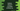# Python program to check if a number is a Niven or Harshad number## Python program to check if a given number is a Niven or Harshad number or not:

A number is called a Niven or Harshad number if the number is divisible by the sum of its digits. For example, 152 is a Niven number because the sum of its digits, 1 + 5 + 2 = 8 can divide 152. Similarly, 156 is also a Harshad or Niven number.

In this post, we will write one program in Python to check if a number is a Niven number or not. It will take the number as input from the user.

### Algorithm to check for a Niven number:

We can use the below algorithm to check for a Niven number:

• Take the number as an input from the user.
• Find the sum of digits of the number.
• Use one while loop to find the sum of digits of the number. This loop will run until the number is greater than 0.
• On each iteration, find the last digit of the number. Add this number to a sum variable. The sum variable should be initialized as 0 at the start of the program.
• Remove the last digit of the number.
• Once the while loop ends, the sum will hold the sum of the digits of the number.
• Check if the sum can divide the number or not.

### Method 1: Python program to check if a number is a Niven number or not:

Let’s write down the python program that checks for a Niven or Harshad number:

``````no = int(input("Enter the number: "))

sum = 0
copy_no = no

while (copy_no):
last_digit = copy_no % 10
sum = sum + last_digit
copy_no //= 10

if no % sum == 0:
print(f'{no} is a Niven number')
else:
print(f'{no} is not a Niven number')``````

Here,

• The number is entered by the user and it is assigned to the no variable.
• It created one variable sum to store the sum of all digits of the number. It is initialized as 0. We also created another variable copy_no to create a copy of the number.
• The while loop is used to find the sum of all digits of the number. This loop runs until the copy_no is greater than zero.
• On each step, it finds the last digit of the number and adds it to the sum variable. It also removes the last digit of the number.
• Once the while loop ends, we are checking if the number can be divided by the sum of digits of the number or not. We are using the modulo operator, % to check the remainder. If it is equal to zero, or if the sum of digits of the number can divide the number, it is a Niven number. Else, it is not an Niven or Harshad number.

If you run this program, it will print output as below:

``````Enter the number: 123
123 is not a Niven number

Enter the number: 63
63 is a Niven number``````

### Method 2: Python program to check if a number is a Niven number or not by using a separate method:

Let’s use a separate method to find if a number is Niven or Harshad. We will call this method and this method will return one boolean value, True or False.

``````def is_niven(no):
sum = 0
copy_no = no

while (copy_no):
last_digit = copy_no % 10
sum = sum + last_digit
copy_no //= 10
return no % sum == 0

if __name__ == "__main__":
no = int(input("Enter the number: "))

if is_niven(no):
print(f'{no} is a Niven number')
else:
print(f'{no} is not a Niven number')``````

So, we created a new method called is_niven to check if a number is Niven or not. It takes one number as its parameter and returns one boolean value. It will print similar output.

``````Enter the number: 63
63 is a Niven number

Enter the number: 64
64 is not a Niven number``````